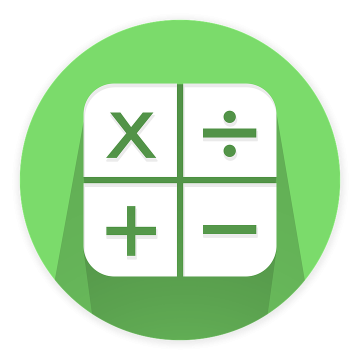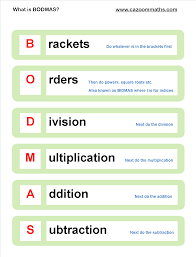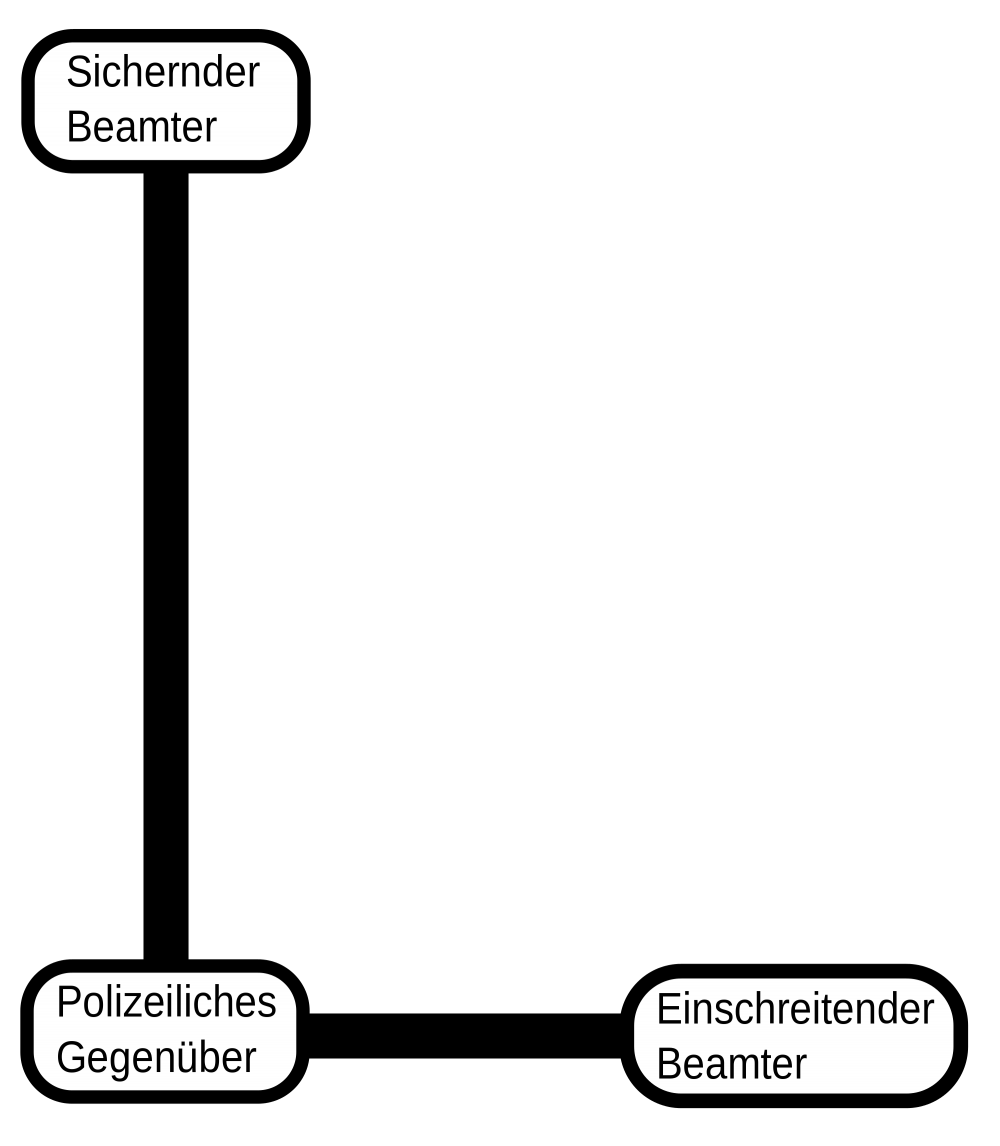BodmasB

O

DM

AS

B=Brackets O=Order D=Division

S=Subtraction

You write it out like this because brackets comes before the order and

that comes before division and multiplication ,addition and subtraction. e.g.

4 (3 + 2)=?

you need to do the operation,or sum,inside the bracket first,3+2,then times the answer by 4.

3+2=5

5 4=20

If you ignored the brackets and did the sum 4 3 + 2= 14

You can see how the brackets make a difference to the answer.Go to the next page and try some questions out..........